The JCM800 power amp follows a long lineage of classic amplifiers that include the Fender Bassman 5F6-A, the Marshall JTM45, the Model 1962 "Bluesbreaker," and the Model 1987 "Plexi." It has substantially more power supply filtering than any of its ancestors, which contributes to a slower, more sustained dynamic as the amp is overdriven. We'll examine the effects here. The amp also derives negative feedback from the 4-ohm output transformer tap instead of the Plexi's 8-ohm tap and doubles the feedback resistor connecting it to the phase inverter. We'll look at these artifacts as well.

#### References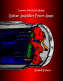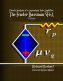## DC Operating Conditions

Here is the power amp and power supply. The voltages are calculated values, not measured values, as described below.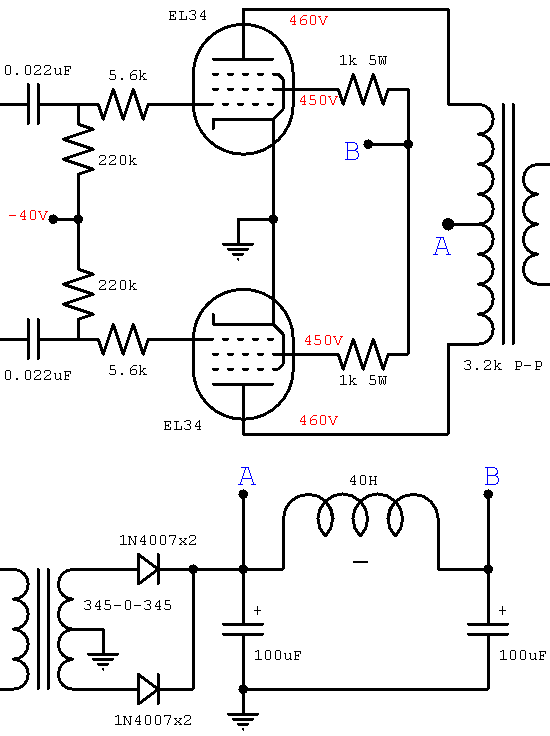The 345-0-345 volt RMS power transformer creates 488 volts DC if there is no load or rectifier loss. If we assume 5 percent sag and less than 5 volts DC loss across the output transformer primary, then we get about 460 volts at the EL34 plates. (For Class AB and a solid state rectifier there is little sag at idle.) A drop of less than 5 volts across the choke gives us at least 455 volts at the top of the 1k Screen resistors. Let's assume about 5mA screen current and see if this holds up under additional scrutiny. That creates a 5-volt drop across the screen resistors to put 450 volts at the screens. The blue curve shown here on the transfer characteristics is our estimate for a 450 volt screen.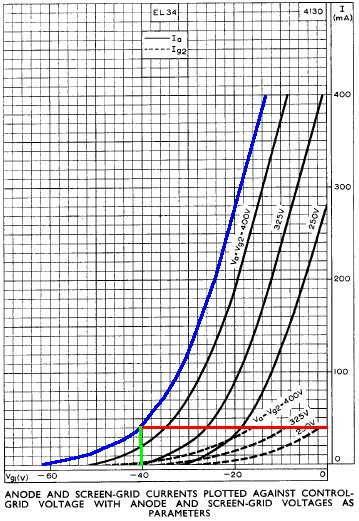Maximum plate dissipation for the EL34 is 25 watts, so if we bias the amp at 70 percent, we get 18 watts, making the idle plate current (18)/(450) = 40mA. This is the red line which intersects the curve at a grid voltage of minus 40 volts. By examining the transfer characteristics for screen current at the bottom of the graph we observe that the idle screen current is well under 10mA, well within the plus-or-minus 20 percent standard of tube amp design, so our original estimate for screen voltage appears reasonable.

## Power Supply Dynamics

According to our LC Ripple Filter Calculator there is 64dB of ripple attenuation for 50-Hertz AC across the choke. For 60-Hertz power it is 67dB. This is substantially more than earlier Marshalls. The JTM45, Bluesbreaker, and Plexi have 20 Henry chokes. The Bassman uses only 10 Henries. Filter capacitor values also increased through the lineage as the price of high-voltage electrolytics dropped. The JTM45 used 32 microfarads on each side of the choke. The JCM800 uses 100 microfarads. The direct result is substantially less hum in the plate and screen supplies. What is often overlooked, however, is the effect of these changes on the dynamics of the power stage when the amp is overdriven.

The resistances in the power transformer, the internal plate resistance of the rectifier tube, and the resistance in the choke cause the DC voltage supplying the power amp screens to sag exponentially. The choke and filter capacitors also interact with one another to cause the voltage to oscillate somewhat, as shown in the following figure.1 The graph shows power supply voltage sag in the Fender Bassman 5F6-A over the first 500 milliseconds after the onset of a full-power signal.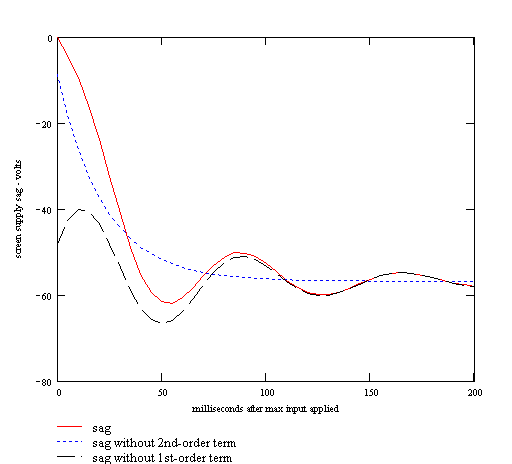The red, solid curve is the actual sag. The blue, dotted curve shows the first order component, whose drop in voltage is determined by the increase in current demand and the characteristics of the power transformer and rectifier. The time period over which the sag takes place depends mostly on the size of the choke and filter capacitors. The JCM800's larger filter reacts more slowly. The black, dashed curve shows the second-order transient, which is caused by the interaction of the choke with the capacitors on either side. This transient is more prominent and decays less quickly in the JCM800.

## Negative Feedback

The JCM800 derives negative feedback from the 4-ohm output transformer tap instead of the Model 1987's 8-ohm tap. It also raises the feedback resistor connecting it to the phase inverter from 47k to 100k. Both factors combine to lower negative feedback by a factor of 3.2

Overdriving the power amp causes output clipping and clamping, which represents a lack of output response to a changing input signal voltage. The output provides the source voltage for negative feedback, so these effects reduce it. This creates more closed-loop gain, which drives the amp further into an overdriven state, producing even more clipping or clamping. The result is that as the amplifier is overdriven, negative feedback from the output transformer accelerates the transition to a more distorted state.

We conclude that the JCM800 has a more sustained, less snappy transition into overdrive compared to its famous ancestors. Combined with the longer time constants in the power supply, the JCM800 performs quite differently when pushed to its limit.

## Full Power Performance

Here are EL34 plate characteristic curves for a 360 volt screen.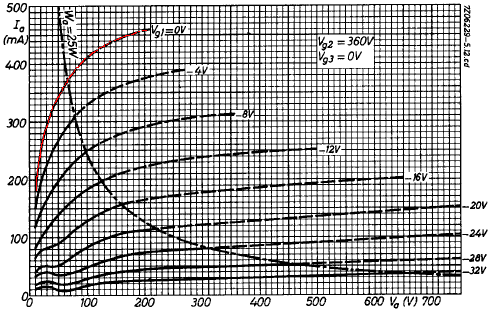To estimate where the load line impacts the zero grid voltage curve (marked in red) we can multiply the plate current by (450/360) raised to the power of 1.5, resulting in a factor of 1.4. We'll ignore all but the top curve, because the other curves need to be redrawn to take into account the tube's cutoff amplification factor.1 Merely increasing the current levels by a factor of 1.4 is an approximation that is valid only for the top curve, where the grid voltage is zero. Here is the redrawn curve in green.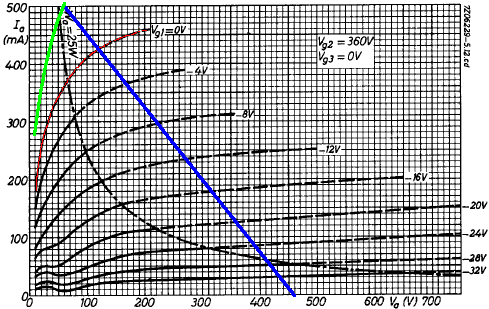The red curve is the zero grid voltage curve for a 360 volt screen. The green curve is our estimate for a 450 volt screen.

The output transformer impedance is 3.2k plate-to-plate. For Class B, or Class AB that is close to Class B, the effective impedance is one fourth this value, or 800 ohms. A plate voltage swing of 400 volts thus creates a plate current swing of 400/800 = 500mA. This is the blue load line in the graph.

We could use the green curve to estimate output power, but the result would be too high by a wide margin. First, it ignores output transformer and speaker losses. Moreover, it assumes no power supply sag, which lowers screen and plate supply voltages and reduces maximum output swing. For the Fender Bassman 5F6-A, for example, full power is about 56 watts without sag and 39 watts at full sag.1 Finally, it doesn't consider increased screen current, which creates a larger voltage drop across the screen resistors.

With a conservative estimate of 60 volts sag at full power, the screen voltage decreases to 390 volts minus some additional DC drop across the choke and an additional drop across the screen resistors, putting us close to the published curve for a 360 volt screen. Plate voltage swing is then 400 - 80 = 320 volts peak and 400mA peak, which creates 128 watts peak and 64 watts RMS for a sinewave. Output transformer loss is in the neighborhood of 10 percent, so we can estimate a maximum power output of 57 watts RMS. The JCM800 Model 2204 is generally classified as a 50-watt amp.

## Input Sensitivity

The input signal amplitude needed to drive the power amp to full power is equal to the DC grid bias, so the JCM800 power amp has an input sensitivity of about 40 volts. This is for the power amp in isolation. What is more important to the designer is the input sensitivity at the input to the phase inverter, which takes into account negative feedback from the 4-ohm output transformer tap. This is what the preamp needs to achieve to drive the amp to full power.

The JCM800 phase inverter is taken directly from the Fender Bassman 5F6-A.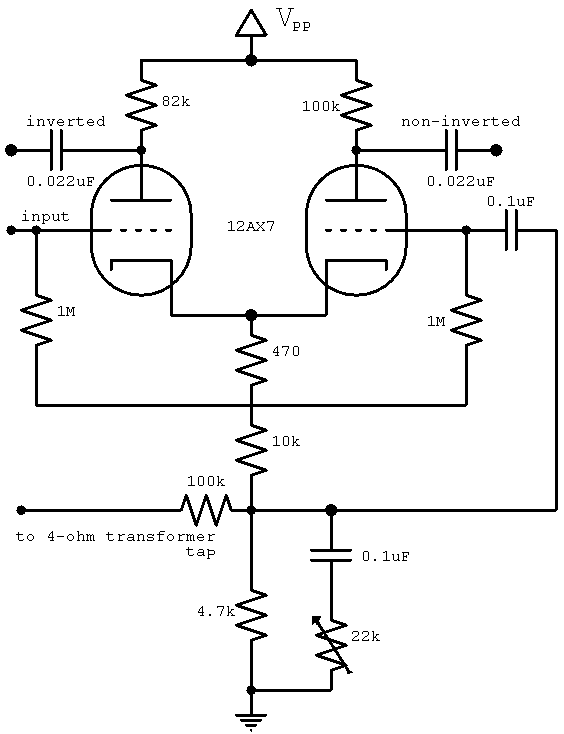The parts values are identical or nearly identical except for the 100k feedback resistor. (Even if the resistor value were identical it could not be compared directly because the Bassman uses different tubes and a different output transformer.) The JCM800 presence control also operates a bit differently than the earlier amps we have mentioned.

According to our Long Tailed Pair Calculator, midrange voltage gain is about 25. This is without the loading of the power amp's 220k grid resistors, but close enough for our purposes here. At full power we get a 400 volt plate voltage swing from a 40 volt grid voltage swing, so the voltage gain from grid to transformer primary is 10. Voltage "gain" from primary to secondary is equal to the square root of the impedance ratio, which for two tubes is 1600/4, for a gain of 0.05. The 100k feedback resistor drives an impedance of about 4k, so the voltage "gain" from transformer tap to phase inverter input is about 4k / (4k + 100k) = 0.04.

Forward gain without feedback is therefore (25)(10)(0.05) = 12.5 and the feedback gain is 0.04. Closed loop gain is thus 12.5 / [1 + (0.04)(12.5)] = 8.3. Negative feedback thus reduces the total voltage gain from about 12.5 to 8.3, representing a loss of only 4dB. This is quite small compared to high-fidelity amplifiers.

The power amp needs a 40 volt input signal amplitude to achieve full power. Without feedback this would mean 40/25 = 1.6 volts at the input to the phase inverter. With feedback we need (1.6)(12.5)/(10) = 2 volts. Our rough estimate for phase inverter input sensitivity is therefore 2 volts.